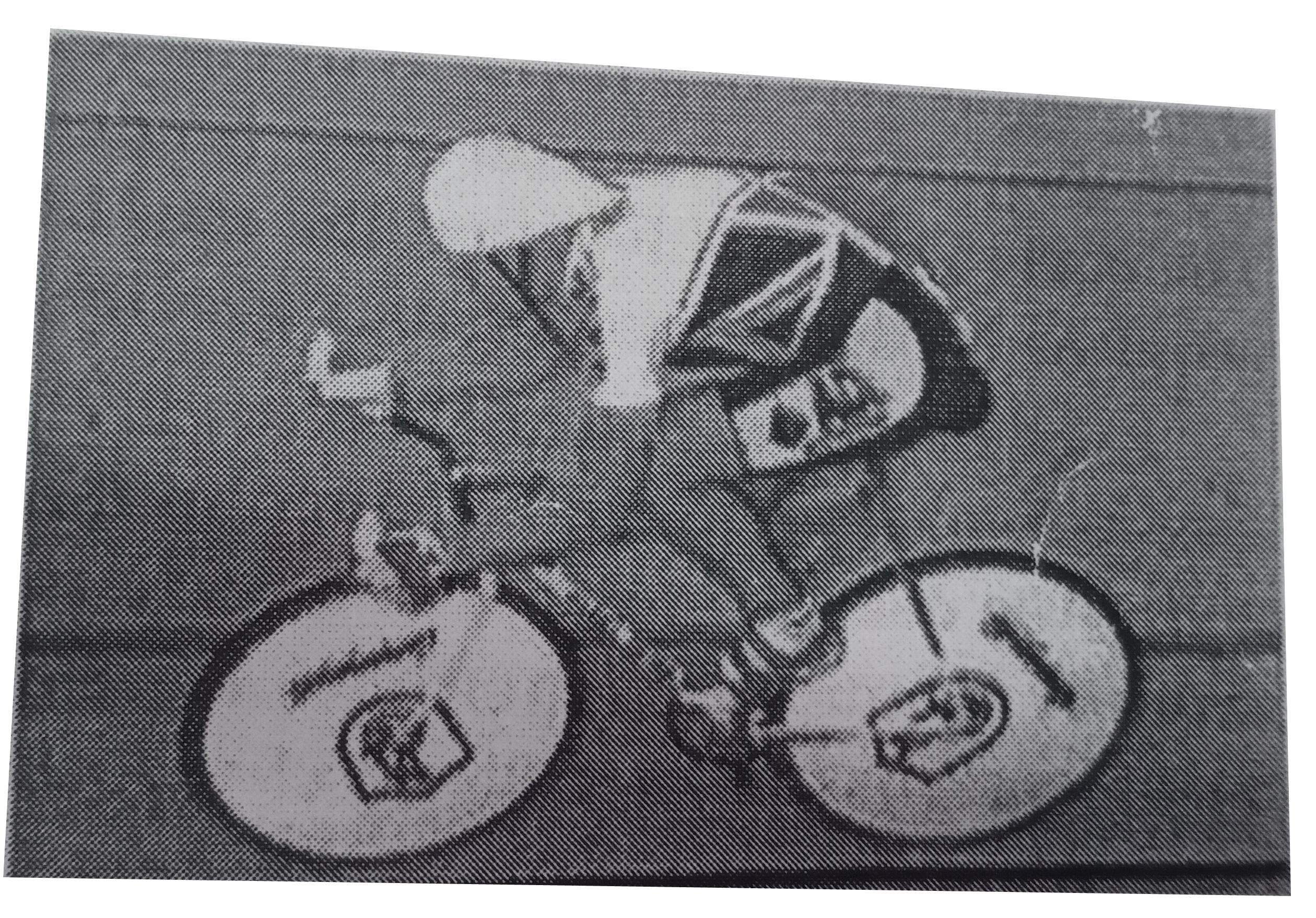Question

Updated on:26/04/2023

# How much work should be done on a bicycle of mass 20 kg to increase its speed from 2ms−1 to 5ms−1 ? (Ignore air resistance and friction).

Text Solution

Solution
We know that whenever work is done, an equal amount of energy is used up. So, the work done in this case will be equal to the change in kinetic energy of bicycle when its speed changes from 2ms1 to 5ms1.
(a) In the first case:
Mass of bicycle, m = 20 kg
And, Speed of bicycle, v=2ms1
So, Kinetic energy, Ek=12mv2
=12×20×(2)2
=10×4
= 40 J
(b) In the second case :
Mass of bicycle, m = 20 kg
And, Speed of bicycle, v=5ms1
So, Kinetic energy, Ek=12mv2
=12×20×(5)2
=10×25
= 250 J
Now, Work done = Change in kinetic energy
= 250 - 40
= 210 J
Thus, the work done is 210 joules.